## Saturday, December 16, 2006

### Bah, Humbug

1.0 Introduction
This post presents an argument I get from Shaikh (1974). During the post (World) War (II) "golden age", the share of profits in U.S. national income stayed fairly constant. As a matter of algebra, an aggregate Cobb-Douglas production function fits the data. One cannot legitimately cite the goodness of such a fit as empirical evidence for the aggregate marginal productivity theory of distribution. The theory has not passed any potentially falsifying empirical test.

Shaikh built on past work in developing his argument, and a body of more recent work has extended and generalized the argument. Why do economists continue to use measures of Total Factor Productivity and Solovian growth theory when they have neither theoretical nor empirical support?

2.0 A Common Version Of Aggregate Neoclassical Theory
Consider the Cobb-Douglas production function: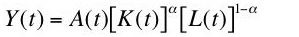(1)
where Y(t) is national income at the indicated time, K(t) is the value of the capital stock, L(t) is labor services, A(t) represents technical progress, and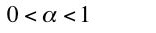(2)
The Cobb-Douglas production function can be written in a per-worker form: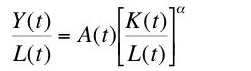(3)
That is, Equation 1 is equivalent to Equation 4:(4)
where y(t) is national income per worker and k(t) is the value of the capital stock per worker. Take natural logarithms of both sides: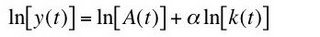(5)
I derive below the Cobb-Douglas production function, in the form of Equation 5, from the assumption that the profit share is constant, independently of whether competitive profit-maximizing firms follow marginal productivity theory or not. This derivation is also independent of whether or not production functions can be aggregated, either across firms or across industries.

Now impose further neoclassical assumptions. By the exploded aggregate neoclassical theory, competitive firms are maximizing profits when the interest rate is equal to the marginal product of capital: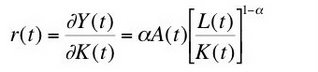(6)
where r(t) is the interest rate. That is, according to neoclassical theory, if the economy’s technology can be represented by an aggregate Cobb-Douglas production function and competitive firms maximize profits, then the share of profits in national income is constant: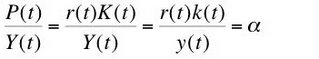(7)
where P(t) is total (accounting) profits.

3.0 Some Accounting Identitites
Begin anew. I start with the accounting identity that national income is the sum of total wages and total profits:(8)
where W(t) is total wages and w(t) is the wage. It is convenient here to do the algebra with quantities expressed per worker: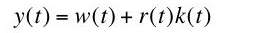(9)
Below, I need the wage share in national income expressed as the difference between unity and the profit share:(10)
Differentiate Equation 9 with respect to time to obtain Equation 11: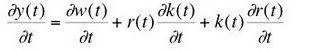(11)
One performs some apparently unmotivated alebraic manipulations on Equation 11 to obtain Equation 12: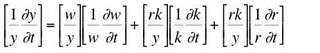(12)
It is worth emphasizing that, so far in this section, all I have been doing is manipulating accounting identities. No additional theoretical or empirical structure has been imposed. I now assume that the profit share is constant, by whatever mechanism brings this constancy about. Equation 12 becomes Equation 13:(13)
Equation 13 expresses a growth-accounting relationship. The left hand side is the rate of growth of national income. The quantities in square brackets on the right hand side are the rate of growth of the wage, the rate of growth of capital per worker, and the rate of growth of the interest rate, respectively.

Take integrals of both sides: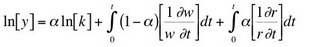(14)
Equation 14 is a Cobb-Douglas production function, in the form of Equation 5, where technical progress is:(15)
So much for Solow's "Nobel" prize.

Update: Originally posted on 5 August 2006. Updated to provide better formatted equations.

Reference
• Shaikh, Anwar (1974). "The Laws of Production and Laws of Algebra: The Humbug Production Function", The Review of Economics and Statistics, V. 56, Iss. 1 (Feb.): pp. 115-120Anonymous said...

«As a matter of algebra, an aggregate Cobb-Douglas production function fits the data.»

I like the «as a matter of algebra» :-).

But it is not just algebra: it is also about defining what "capital" etc. mean, as you know better than most.

«One cannot legitimately cite the goodness of such a fit as empirical evidence for the aggregate marginal productivity theory of distribution.»

But it can be cited as evidence that if the data can be made to fit the theory, by adopting suitable definitions for the data :-).

«The theory has not passed any potentially falsifying empirical test.»

I don't like this: this is vulgar popperianism, and who cares? To me the purpose of political economics is insight, not theory, never mind a mechanistic theory that can/cannot be falsified.

But I am not sure even of that... The problem with economics is that whatever laws or empirical relationships you find one decade, they will be different in a couple of decades.

Because the system under observation evolves *quickly*, often as a consequence of the observation.

«Why do economists continue to use measures of Total Factor Productivity and Solovian growth theory when they have neither theoretical nor empirical support?»

Because it helps their careers? Because it conveniently begs away a number of difficult questions? :-)

BTW, nice derivation of some amusing identities. And nice find of that article which has that nice "humbug production function" in the title.Anonymous said...

«One cannot legitimately cite the goodness of such a fit as empirical evidence for the aggregate marginal productivity theory of distribution.»

No, but what it means is that IF an economy can be usefully represented by an aggregate production function then that function is Cobb Douglas (or some close version of it) as that's the only kind consistent with Kaldor's stylized facts and the data (constant shares, constant interest rates, balanced growth path with steady growth - let's leave aside for the moment how true these facts really are (Chuck Jones has some stuff on that)).

But speaking of interest rates and falsifiability (however you spell that). Your model doesn't even determine interest rates. How can it be falsifiable itself? What stylized facts does it fit? Where is there any empirical evidence that I should take it as a useful representation of reality?
In trying to answer those questions one might get some inkling of why some people still use APFs. That and because a lot of past empirical research in development and growth says that physical capital, fixed or circulating, isn't as important as we thought. Hence people feel like they're wasting their time arguing about what exactly the proper definition of capital should be. Of course you can spin that another way.

Robert Vienneau said...

I suppose I should have acknowledged comments before.

I can see why some object to some interpretations of Popper. Popper said that, in some sense, falsifiability is not a logical property of theories, but a sociological property of communities who hold those theories. If mainstream economists were serious, references to Shaikh and Franklin Fisher would be commonplace in papers that use aggregate production functions and Total Factor Productivity accounting. But they are not.

I think of aggregation arguments and cambridge capital controversy arguments about the meaning of capital as parallel to the argument in the post. I would not call the argument in the post a "model". I don't know why Radek thinks the failure of those equations to describe a mechanism by which interest is determined an objection. (I'll let you know when I present an alternate "model".)Anonymous said...

I don't know why you're down on Total Factor Productivity. Yeah, you can get it from the Solow model as the residual, but you can also get it from you equation (15), without assuming anything about Aggregate Production functions - just plug in 0 for dr/dt and g for dw/dt and you got your A(t) which is TFP. The thing is, either way you get pretty much the same answer. Hmmm, strange.